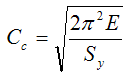## Posts

Showing posts from August, 2011

### Improve math skills of your kids - Learn step-by-step arithmetic from Math games

Math: Unknown - Step-by-step math calculation game for iOS.

Math: Unknown is much more than a math game. It is a step-by-step math calculation game which will teach users how to calculate in the correct order rather than just asking only the final calculated results.

The app consists of four basic arithmetic operations which are addition, subtraction, multiplication and division. In order to get started, users who are new to arithmetic can learn from animated calculation guides showing step-by-step procedures of solving each type of operation. It is also helpful for experienced users as a quick reference.

Generally, addition and subtraction may be difficult for users who just start learning math especially when questions require carrying or borrowing (also called regrouping). The app helps users to visualize the process of carrying and borrowing in the way it will be done on paper. Once users understand how these operations work, they are ready to learn multiplication and division.

For most students, division is considered as the most difficult arithmetic operation to solve. It is a common area of struggle since it requires prior knowledge of both multiplication and subtraction. To help users understand division, the app uses long division to teach all calculation procedures. Relevant multiplication table will be shown beside the question. Users will have to pick a number from the table which go into the dividend. Multiplication of selected number and divisor is automatically calculated, but the users have to do subtraction and drop down the next digit themselves. Learning whole calculation processes will make them master it in no time.

Math: Unknown is a helpful app for students who seriously want to improve arithmetic calculation skills.

### Column Design (Part 3)Let's continue from the previous post ...
The criteria to select whether we should use the Euler formula or the J. B. Johnson formula to calculate for the critical load (Pcr) of the column is related to the value of the actual slenderness ratio or column constant, Cc. It is defined as
where: E = Modulus of elasticity of the material of the column Sy = Yield strength of the column material
The use of the above column constant (Cc) is as follows, Determine the length and end fixity of the columnDefine the value of the constant (K) according to the type of end fixityCompute the effective length (Le) from Le = KLFrom the cross section shape and dimensions, compute the radius of gyration (r) from r = sqrt(I/A)Compute the slenderness ratio from Slenderness ratio = Le/r_min = KL/r_minFrom the material of the column, compute the column constant (Cc) as per the above formulaCheck whether KL/r > Cc?If yes, the column is Long: Use the Euler formulaIf no, the column is Short: Use the J. B. …

### Column Design (Part 2)From Column Design (Part 1), we know that a column will tend to buckle  about the axis for which the radius of gyration (r) and the moment of inertia (I) are minimum. Another important parameter for column design is the effective length (Le) of the column. The effective length is defined as

Le = KL

where:
L = Actual length of the column between its supports
K = Constant value dependent on the end fixity of the column as following.
A pinned-end column is guided so that the end of the column cannot sway from side to side, but it can rotate with no resistance at the end.A fixed-end column is held against rotation at the support. The higher constant value of K as shown as the "practical values" in the above table is recommended because in reality it is particularly difficult to achieve a true fixed-end column because of lacking of rigidity of the support.
The slenderness ratio is the ratio of the effective length of the column to the least radius of gyration.
Slenderness ratio = Le…